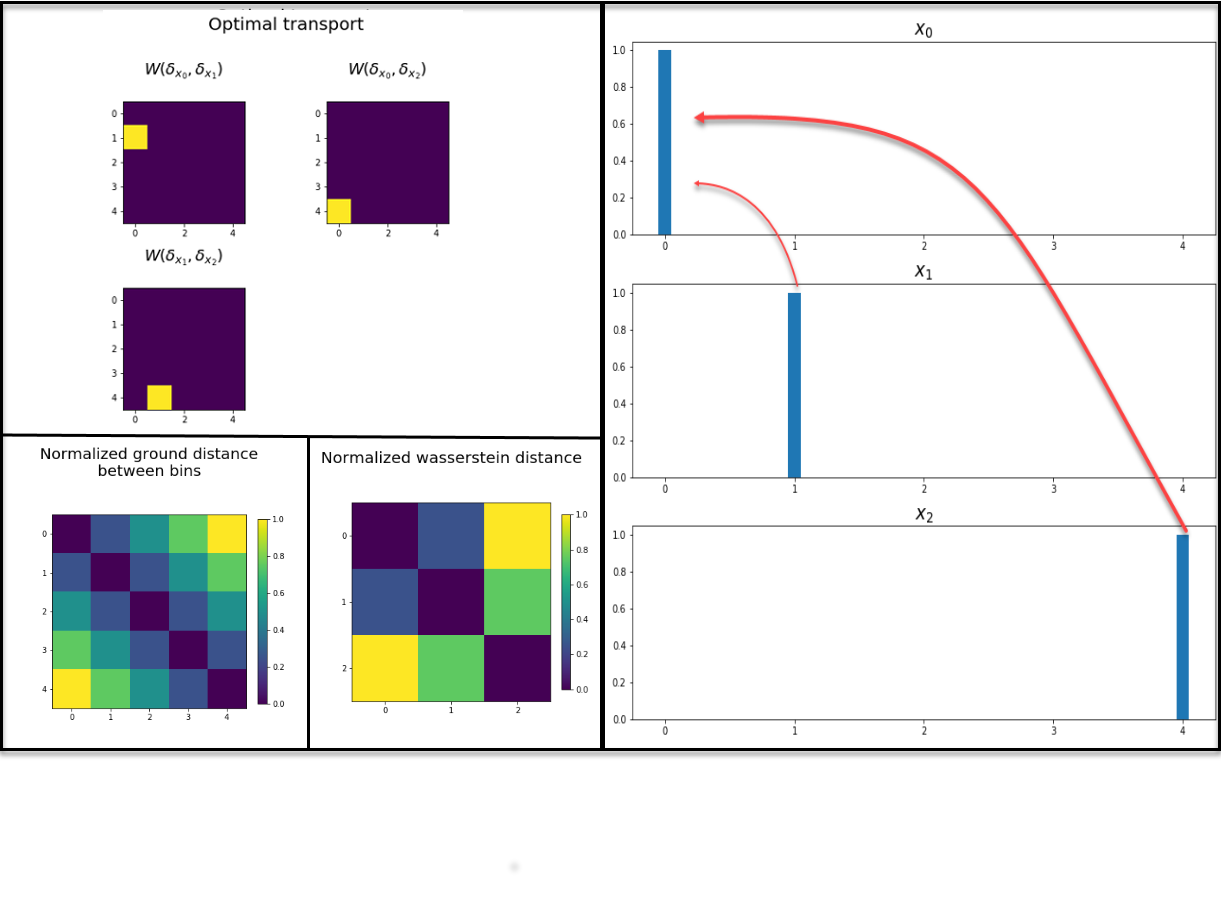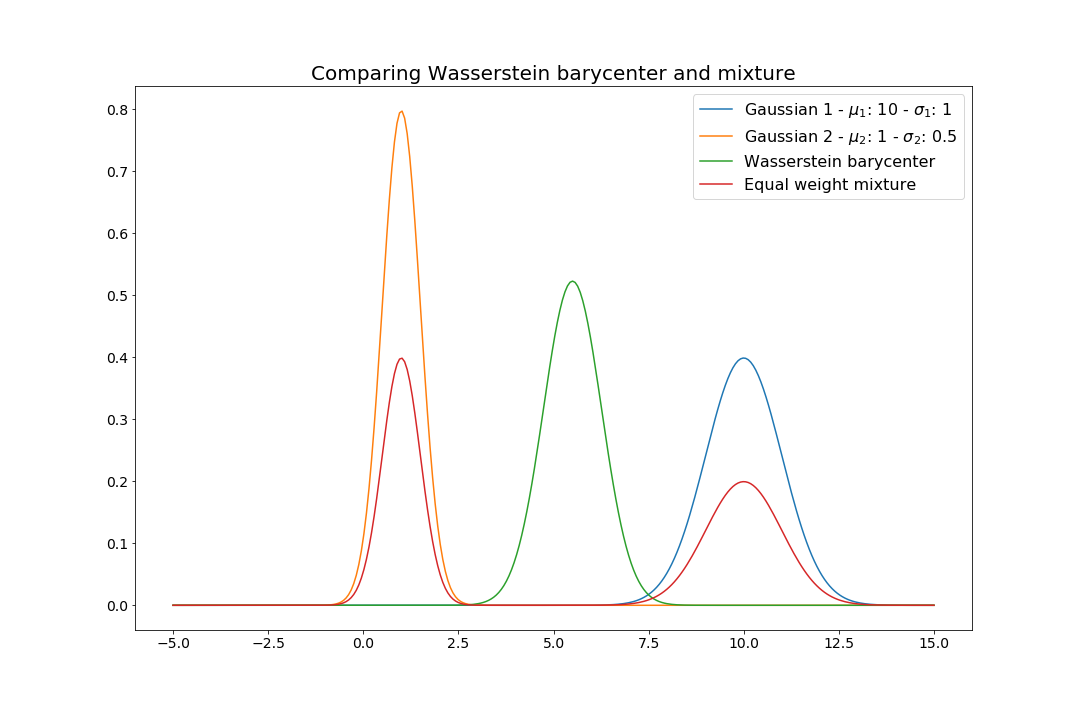# Introduction

The Wasserstein distance and optimal transport theory were first studied by the French mathematician Gaspard Monge in the 18th entury. Since that time, the field has been revisited by many illustrious mathematicians like Leonid Kantorovich in the 20th century and more recently by the Fields medalist Cédric Villani.

In addition to these considerable theoretical advances, the method has also benefited from important advances in numerical computations and algorithms which have made it amenable to real large scale problems. Consequently, optimal transport has been applied successfully to various contexts such as regularization in statistical learning, distributionally robust optimization, image retrieval and economics.

This post focuses on the basic concepts underlying the Wasserstein distance, detailing some of its interesting properties as well as practical numerical methods. It shies away from the often math-heavy formulations and tries to focus on the important practical questions to build intuition.

# Basic formalism and notation

Given an arbitrary base set $$\Omega \subset \mathbb{R}^d$$, $$\mathcal{M}(\Omega)$$ denotes the set of probability measures on $$\Omega$$ and $$m(\mu,\nu)$$ represents the set of joint distributions with marginals $$\mu$$ and $$\nu$$ in $$\mathcal{M}(\Omega)$$. Using some distance $$D : \Omega \times \Omega \rightarrow \mathbb{R}_+$$ such as the $$l_p$$ norms with $$p\in \mathbb{N}$$, the $$p$$-Wasserstein distance is then defined as the solution to the following optimization problem:

$W_p(\mu,\nu) = \displaystyle \inf_{\Pi \in m(\mu,\nu) } \left( \int_{\Omega} \int_{\Omega} D(x,y)^p d\Pi(x,y) \right)^{\frac{1}{p}}.$

A particular, but usefull case is the situation where we consider only discrete measures. In that case, we have a finite set of points/atoms $$X=\{x_1,\cdots,x_n \}$$ and $$Y=\{y_1,\cdots,y_m \}$$ and the measures take the form $$\mu = \sum_{i=1}^n \delta_{x_i} a_i$$ and $$\nu= \sum_{j=1}^m \delta_{y_j} b_i$$ for $$a \in \Delta_n$$ and $$b \in \Delta_m$$ where $$\Delta_l=\{ u \in \mathbb{R}^l_+: \sum_i u_i =1 \}$$ is the simplex and $$\delta_{x_i}$$ is a dirac measure putting all weight on $$x_i$$.

In that case, the Wasserstein distance (to the $$p$$th power) takes on the much simpler form of a linear transportation problem, which we write explicitely in terms of the marginal probabilites $$a\in \Delta^n$$ and $$b \in \Delta^m$$:

\begin{align} W_p^p(a,b) = \min_{\pi} & \sum_{i=1}^n \sum_{j=1}^m \pi_{ij} D(x_i,y_j)^p \\ \text{s.t.} & \sum_{i=1}^n \pi_{ij} = b_j , \forall j\\ & \sum_{j=1}^m \pi_{ij} = a_i , \forall i\\ & \pi_{ij} \geq 0, \forall (i,j) \end{align}

The problem has a simple intuitive meaning as the minimum amount of work required to transform one distribution into the other. Indeed, when $$p=1$$ this is called the Earth Mover Distance and can be though of as moving dirt from high valued bins of one distribution to throughs in another. Each time, we consider the physical distance between the bins and prioritize bins that are close to one another according to the ground distance $$D$$.

# Properties and rationale

Like other distances, it is non-negative, symmetric, subadditive and 0 only if the 2 measures are identical. It also offers interesting advantages over standard distances like the Euclidean distance. For instance, we notice that $$n$$ and $$m$$ can be different, which implies we can compare distributions with a different number of bins.1

Together with an adequate choice of ground distance $$D: \Omega \times \Omega \rightarrow \mathbb{R}_+$$, the Wasserstein distance can capture important local properties of the problem. Each bin of a distribution is compared to every other bin of the other distribution, but closer bins are less penalized that ones that are farther. For images, this might help capture the local correlation in pixel intensity2. In contrast, using the raw Euclidean distance would require having 2 histograms with exactly the same number of bins/points/atoms and comparing values bin by bin.

As shown in the important Rubner et al. paper, these properties help the Wasserstein distance better capture perceived similarities between distributions than the standard Euclidean distance. The authors show that the Wasserstein distance remains superior even for distances induced by quadratic norms $$D(x,y) = ||K (x-y)||_2$$, which do not suffer from the bin by bin comparison of the standard Euclidean distance for some symmetric positive definite matrix $$K \in \mathbb{R}^{d\times d}$$.

# A small example

Consider the following vectors $$x_0, x_1, x_2 \in \mathbb{R}^5$$, represented in the figure below:

Further assume that each of the 5 dimensions represents a position on a line so that we can determine that the distance between positions/indices 0 and 4 is $$D(0,4)=|0-4|$$, which is four times $$D(0,1)=|1-0|$$, the distance between positions 0 and 1.

### Euclidean distance

As the figure on the right illustrates, all 3 vectors are all equally dissimilar with a maximal Euclidean distance of $$\sqrt{2}$$ since they differ by 1 at exactly 2 positions. Hence the normalized distance is 1 off the diagonal and 0 otherwise.

### Wasserstein distance

The figure below illustrates that the result is different when computing the Wasserstein distance between the 3 discrete measures $$\mu_i = \delta_{j_i}, i=0,\cdots,2$$ placing unit mass at $$j_0 =0, j_1= 1, j_2=4$$. Indeed, with that choice of distance, $$x_0$$ and $$x_1$$ are much closer than $$x_0$$ and $$x_2$$ and $$x_1$$ and $$x_2$$3.Computing the Wasserstein distance between three discrete measures with a single atom. Although the ground distance is symmetric accross both diagonals, the Wasserstein distance reflects the intuitive fact that moving 1 unit of mass from 4 to 1 is costlier than moving it from 4 to 0. The top left and right quadrants illustrate the optimal transport plan while the central bottom square illustrates the actual normalized Wasserstein distance.

# Wasserstein barycenters

It is also interesting to study the barycenters generated by the Wasserstein distance. Following our intuition based on the usual Euclidean norm and the fact that $$\sum_{i=1}^N \frac{x_i}{N}\in \arg\max_u \sum_{i=1}^N \frac{1}{N}||x_i-u ||_2$$ (which is one of the key results underlying the $$k$$-means algorithm for instance), we can think of barycenters as centroids or representative of a set of objects (measures in this case). More formally, given a set of $$N$$ distributions $$\{\nu_i\}_{i=1}^N$$, the Wasserstein barycenter is defined as the measure that minimizes the following problem:

$\mu^* = \arg\min_{\mu} \displaystyle \frac{1}{N} \sum_{i=1}^N W_p^p(\mu,\nu_i)$

The following sections detail two important cases in which we can find such solutions either numerically or analytically.

### Analytical solution for Gaussian distributions

In the specific case where $$\nu_i \sim \mathcal{N}(m_i, \Sigma_i)$$ are all Gaussian, Agueh and Carlier show that $$\mu^* \sim \mathcal{N}(\sum_{i=1}^N\frac{m_i}{N} , \hat{\Sigma})$$, where $$\hat{\Sigma}$$ is a semidefinite symmetric matrix satisfying $$\hat{\Sigma} = \frac{1}{N} \sum_{i=1}^N (\hat{\Sigma}^{1/2} \Sigma_i \hat{\Sigma}^{1/2})^{1/2}$$. In other words, the barycenter remains Gaussian with a mean given by the arithmetic average of the mean of the $$\{\nu_i\}$$ and the covariance matrix satisfies a non-linear equation.

As illustrated in the figure below, this contrasts with the mixture distribution $$\sum_{i=1}^N \frac{1}{N}\mathcal{N}(m_i, \Sigma_i)$$ which is in general not Gaussian. This shape preserving property has numerous useful applications in image processing, particularly for texture interpolation and texture mixing.Comparing Wasserstein barycenter and mixture for Gaussian distributions.

The Wasserstein barycenter in the preceding example was computed using the excellent $$\texttt{ot}$$ Python module. For more details and in-depth examples, check out the documentation.

### Numerical methods for discrete measures

Another practically important situation, which is discussed in details in Cuturi and Doucet, is the case where we wish to find the barycenter of a set of arbitrary discrete measures that have the same support $$X=\{x_1,\cdots,x_n\}$$. In order to obtain the Wasserstein barycenter for such a set of measures, we may use a simple projected subgradient method where we consider the average distance between the incumbent barycenter and one of the $$N$$ measures $$\{\nu_k\}_{k=1}^N$$ at each iteration.

More specifically, we formulate the problem in terms of the right hand side marginal probabilities $$a \in \Delta^n$$ and $$b_k \in \Delta^n$$ and solve the following problem:

$\min_{a \in \Delta} \displaystyle \frac{1}{N} \sum_{k=1}^N W_p^p(a,b_k)$

We let $$f(a) = \sum_{k=1}^N \frac{1}{N} f_k(a)$$ where $$f_k(a) = W_p^p(a,b_k)$$ and set $$D_{ij}^k=D(a_i,b_{j}^k)^p$$. We then observe that the dual of $$f_k(a)$$ is

\begin{align} \max_{\alpha \in \mathbb{R}^n,\beta \in \mathbb{R}^n } & \alpha^{\top} a + \beta^{\top} b_k \\ \text{s.t.} \; & \alpha_i + \beta_j \leq D_{ij}^k , \forall (i,j)\\ \end{align}

We note that if $$a$$ and $$b_k$$ are in the simplex, both primal and dual problems are feasible and bounded so that both problems have the same finite optimal objective value. We can then show that $$f(a)$$ is convex 4 and $${\alpha} = \sum_{k=1}^{N}\frac{1}{N} \alpha_{0k}$$ is a subgradient of $$f$$ at $$a$$ where $$(\alpha_{0k},\beta_{0k}) \in \arg\max_{\alpha,\beta} \alpha^{\top} a_0 + \beta^{\top} b_k: \alpha_i + \beta_j \leq D_{ij}^k , \forall (i,j)$$.

Indeed, $$f_k(a_0) = \alpha_{0k}^{\top} a_0 + \beta_{0k}^{\top}b_k$$ by strong duality. We also have $$f_k(a) \geq \alpha_{0k}^{\top}a + \beta_{0k}^{\top} b_k$$ for any $$a \in \Delta$$ by weak duality. It follows that $$f_k(a_0) + \alpha_{0k}^{\top} (a-a_0) \leq f_k(a), \forall a \in \Delta$$ and thus $$\sum_{k=1}^N \frac{1}{N} f_k(a_0) + \sum_{k=1}^N \frac{1}{N} \alpha_{0k}^{\top}(a-a_0) \leq \sum_{k=1}^N \frac{1}{N} f_k(a)$$.

We can therefore use the step $$a_{l+1} = P_{\Delta}(a_l - \eta {\alpha}) = \arg\min_{u \in \Delta} || (a_l - \eta {\alpha}) -u||_2$$, where $$P_{\Delta}$$ is the projection onto the simplex and $$\eta>0$$ is some predetermined step length.

However, this projected subgradient is only meant for presentation purposes. Indeed, each iteration with a fixed $$a_l$$ requires computing $$k$$ Wasserstein distances and hence solving $$k$$ (primal) linear programs with $$O(n^2)$$ variables or $$k$$ (dual) linear programs with $$O(n^2)$$ constraints.

There exists other more sophisticated method such as a multimarginal linear problem where the number of variables is $$O(n^N)$$ and is therefore intractable for most real problems. More importantly, an extremelly efficient fixed point algorithm can be used to solve an approximate regularized version. Details on this Sinkhorn algorithm as well as countless other details and variations are given in the Peyré and Cuturi book.

# Conclusion

This post illustrates that the Wasserstein distance is an extremelly powerful and versatile concept. Its locally aware shape preserving properties can be used for numerous applications and it benefits from various sophisticated numerical algorithms.

Regardless of these important advances, the field of optimal transport theory is extremelly rich and dynamic. As of 2020, many theoretical and practical research initiatives are underway and numerous applications are still being developped.

1. Of course, we can always take $$l=\max\{n,m\}$$ and consider vectors of dimension $$l$$ padded with zeros. However, this will increase the dimension of one of the vectors, which might be undesirable if $$l$$ is very large.

2. A greyscale pixel image can be seen as a discrete 2 dimensional distribution where the bins represent pixels (points in a 2D grid) and the density is given by the normalized grey intensity in $$[0,1]$$. If we want to compare images with resolution (say) 30 X 30, we might then want to evaluate the intensity of the 2 images at pixels $$(2,3)$$, $$(3,4)$$ and $$(15,15)$$. Using the l1 norm as ground distance, $$(2,3)$$, $$(3,4)$$ are at a distance of $$|3-4| + |4-3| = 2$$ while $$(2,3)$$ and $$(15,15)$$ are at a distance of $$|15-2| + |15-3| = 25$$. Moving intensity from pixel $$(2,3)$$ to $$(15,15)$$ is therefore much costlier in the Wasserstein sense than moving it from $$(2,3)$$ to $$(3,4)$$, which helps capture the local correlation intensity in images.

3. In this simple case, the Wasserstein distance corresponds exactly to the basic ground distance since histograms only have a single bin of mass 1, but this is not the case in general.

4. $$f(a)$$ is convex since duality reveals it is the maximum of a (finite) collection of affine functions of $$a$$. See Boyd and Vandenberghe for more details.